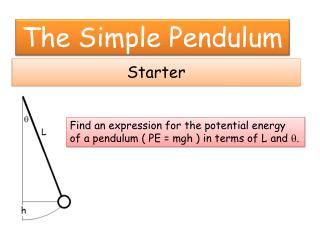DownloadDownload PresentationStarter

# Starter

Download Presentation## Starter

- - - - - - - - - - - - - - - - - - - - - - - - - - - E N D - - - - - - - - - - - - - - - - - - - - - - - - - - -
##### Presentation Transcript

1. Starter The Simple Pendulum Find an expression for the potential energy of a pendulum ( PE = mgh ) in terms of L and q.

2. Practice PE = mgL (1-cosq).

3. Application – Energy in a Pendulum If a pendulum is released from rest and the velocity measured at the lowest point: mgL(1-cosq) = (1/2)mv2 or (1)

4. Design an experiment to verify the energy equation with at least 10 different trials. All of the lab goes in your science notebook. Measure and record L and q and then release the ball from rest. Measure and record the velocity at the bottom of the swing using the photogate timer. This is vexp 3. Calculate the theoretical velocity. vtheor = 4. Compare vexp and vtheorwith a percent difference.

5. Setting Up Labquest Photogate Mode: Photogate timing Photogate mode: GATE Length of Object: .0254

6. Questions If you used a longer string but the same angle, how would the velocity change? If you did this experiment on the moon, how would the velocities change?

7. Lab Checklist Starter Data table Calculation Samples 2 Questions Summary